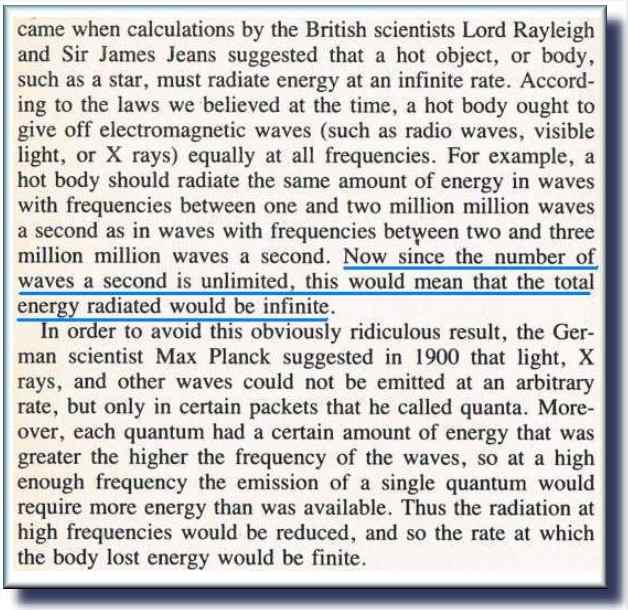Xeno and Planck Jonathan Ainsley Bain

 Xeno’s Paradox: The first step to solving quantum gravity (from Ancient Greece) Achilles and a tortoise are to race. Achilles offers the tortoise a head start. At a certain point in time {t=1} Achilles is half-way to the tortoise, but at this point the tortoise has ambled a few steps ahead. At the next point in time that we measure {t=2} Achilles is half way to where the tortoise ambled, but at this point the tortoise again has advanced somewhat. At {t=3} Achilles is once more half way to the advanced point while the tortoises makes slow progress. When {t=4} Achilles tries to catch up to the tortoises’ progress, but the tortoise has loped forward a bit. At {t=5} Achilles once more gets closer, but the tortoise has again crept forward a little bit. And so the race continues into infinity with the tortoise not ever being overtaken. Because each time that Achilles strides half-way to the tortoise, the tortoise takes a little step forward…

 The Ultraviolet Catastrophe The second step to solving quantum gravity (from Hawking's 'Brief History of Time')The similarity between these two ideas is striking. Planck solved the problem of the Ultraviolet catastrophe by eliminating infinity from the argument and establishing a finite amount of energy, the quanta, which became known as Planck’s constant. The way to solve Xeno’s paradox is just the same. Time cannot be infinitely divided. Eventually we reach a point where time behaves a bit like a particle. At this point the distance covered must finally be greater by Achilles than the tortoise. And so, at last we can rescue Achilles’ reputation and he overtakes the tortoise. This can be demonstrated by a computer program which uses an event-driven timer that moves objects across the screen at intervals. The interval between events can be set to one second, half a second, or less. But eventually we cannot keep making the interval infinitely smaller. At some point the computer processor reaches its limit and time must be quantized. At this quantized point, the amount of distance covered by the object (velocity) comes into account and Achilles can overtake the tortoise. We could solve Xeno’s paradox by making space into quanta too. So that even if time could be infinitely divided, the finite quanta of space would prevent us from doing so. Do finite space quanta and finite time quanta represent the same point of view? Infinite divisibility of either space or time results in error, so both must have finiteness as a property. They both must exist as quanta. One of them must be quantized in a primary manner in order to compute. Either velocity is distance per time unit; or velocity is time elapsed for a quantum of distance. Both give correct results. But the denominator cannot be zero from either point of view. The ultraviolet catastrophe was solved by the same measure. By assuming an infinite number of waves per second, we essentially end up with a wavelength of zero. To avoid all of these catastrophes we have to simply ask the question: ‘Why do we assume an infinite amount of anything?’ Simply put, if infinity were applicable we end up with a problem, so infinity is not an applicable property. This tells us two main ideas. Firstly, that space, time, energy, mass, wavelength, and frequency, etc are all in finite quanta. Secondly, we must have obtained the concept of infinity from beyond the physical world. For we could not have learnt it from the physical world, as whenever we apply it to the physical world we end up with contradictions like Achilles not being able to overtake a tortoise; or with ridiculous notions like infinite energy at any one point. Once more the same conclusion as the previous chapter has been reached. We cannot have learnt from the physical world that infinity exists, because nowhere in the physical is it applicable. And yet we know that it exists for the simple reason that we all know what the word means. Thus it is another a priori Platonic form that precludes the notion of tabula rasa. Certain knowledge is in us inherently. The ultraviolet catastrophe, and Xeno’s paradox are essentially the same error. Both occur for lack of quantization. Both occur because calculus assumes infinity. But in reality, ‘infinity’ is never completely infinite. It only appears thus. Real time computation demands quantum time..! Continues Here : < The third step to solving quantum gravity >